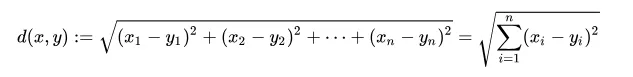欢迎访问我的网站，希望内容对您有用，感兴趣的可以加入免费知识星球。

# 欧几里得距离

4年前 (2019-11-30) 9547次浏览

## 软硬件环境

• ubuntu 18.04 64bit
• numpy 1.12.1

## 前言## 代码实现

``````#!/usr/bin/env python
# -*- coding: utf-8 -*-
# @Date    : 2018-08-17 16:31:07
# @Author  : xugaoxiang (xugx.ai@gmail.com)
# @Version : 1.0.0

import numpy as np

def get_edclidean_distance(vect1,vect2):
dist = np.sqrt(np.sum(np.square(vect1 - vect2)))
# 或者用numpy内建方法
# dist = numpy.linalg.norm(vect1 - vect2)
return dist

if __name__ == '__main__':

vect1 = np.array([1,2,3])
vect2 = np.array([4,5,6])

print(get_edclidean_distance(vect1, vect2))
``````

``5.19615242271``